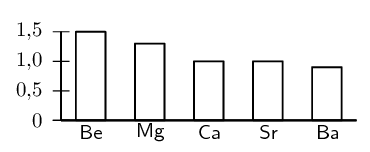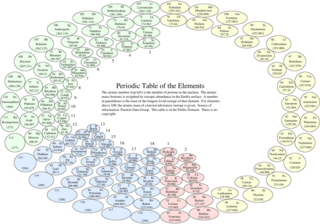Home Practice
For learners and parents For teachers and schools
Textbooks
Full catalogue
Pricing SupportLog in

We think you are located in United States. Is this correct?

# 5.2 Chemical properties of the groups

## 5.2 Chemical properties of the groups (ESABP)

In some groups, the elements display very similar chemical properties and some of the groups are even given special names to identify them. The characteristics of each group are mostly determined by the electron configuration of the atoms of the elements in the group. The names of the groups are summarised in Figure 5.3

A few points to note about the groups are:

• Although hydrogen appears in group 1, it is not an alkali metal.

• Group 15 elements are sometimes called the pnictogens.

• Group 16 elements are sometimes known as the chalcogens.

• The halogens and the alkali metals are very reactive groups.

• The noble gases are inert (unreactive).

The following diagram illustrates some of the key trends in the groups of the periodic table:

Table 5.2 summarises the patterns or trends in the properties of the elements in group 1. Similar trends are observed for the elements in the other groups of the periodic table. We can use the information in Table 5.2 to predict the chemical properties of unfamiliar elements. For example, given the element Francium ($$\text{Fr}$$) we can say that its electronic structure will be $$[\text{Rn}]7\text{s}^1$$, it will have a lower first ionisation energy than caesium ($$\text{Cs}$$).

One general trend that is not shown is the melting and boiling points. For the metals (groups 1 to 13) the melting and boiling points increase as you go up the group. For the non-metals the melting and boling points decrease as you go up the group.

You should also recall from chapter Chapter 2 that the metals are found on the left of the periodic table, non-metals are on the right and metalloids are found on the zig-zag line that starts at boron.

 Element $$_{3}^{7}\text{Li}$$ $$_{11}^{23}\text{Na}$$ $$_{19}^{39}\text{K}$$ $$_{37}^{86}\text{Rb}$$ $$_{55}^{133}\text{Cs}$$ Electron structure $$[\text{He}]2\text{s}^1$$ $$[\text{Ne}]3\text{s}^1$$ $$[\text{Ar}]4\text{s}^1$$ $$[\text{Kr}]4\text{s}^1$$ $$[\text{Xe}]5\text{s}^1$$ Group 1 chlorides $$\text{LiCl}$$ $$\text{NaCl}$$ $$\text{KCl}$$ $$\text{RbCl}$$ $$\text{CsCl}$$ Group 1 elements all form halogen compounds in a 1:1 ratio Group 1 oxides $$\text{Li}_{2}\text{O}$$ $$\text{Na}_{2}\text{O}$$ $$\text{K}_{2}\text{O}$$ $$\text{Rb}_{2}\text{O}$$ $$\text{Cs}_{2}\text{O}$$ Group 1 elements all form oxides in a 2:1 ratio Atomic radius Increases as you move down the group. First ionisation energy Decreases as you move down the group. Electronegativity Decreases as you move down the group. Melting and boiling point Decreases as you move down the group. Density Increases as you move down the group.

Table 5.2: Summary of the trends in group 1

## Groups in the periodic table

Textbook Exercise 5.2

Use Table 5.2 and Figure 5.4 to help you produce similar tables for group 2 and group 17.

Solution not yet available.

The following two elements are given. Compare these elements in terms of the following properties. Explain the differences in each case. $$_{12}^{24}\text{Mg}$$ and $$_{20}^{40}\text{Ca}$$.

1. Size of the atom (atomic radius)

2. Electronegativity

3. First ionisation energy

4. Boiling point

Solution not yet available.

Study the following graph and explain the trend in electronegativity of the group 2 elements.Solution not yet available.

Refer to the elements listed below:

• Lithium ($$\text{Li}$$)

• Chlorine ($$\text{Cl}$$)

• Magnesium ($$\text{Mg}$$)

• Neon ($$\text{Ne}$$)

• Oxygen ($$\text{O}$$)

• Calcium ($$\text{Ca}$$)

• Carbon ($$\text{C}$$)

Which of the elements listed above:

1. belongs to Group 1

2. is a halogen

3. is a noble gas

4. is an alkali metal

5. has an atomic number of 12

6. has four neutrons in the nucleus of its atoms

7. contains electrons in the 4th energy level

8. has all its energy orbitals full

9. will have chemical properties that are most similar

Solution not yet available.

## Inventing your own periodic table

You are the official chemist for the planet Zog. You have discovered all the same elements that we have here on Earth, but you don't have a periodic table. The citizens of Zog want to know how all these elements relate to each other. How would you invent the periodic table? Think about how you would organise the data that you have and what properties you would include. Do not simply copy Mendeleev's ideas, be creative and come up with some of your own. Research other forms of the periodic table and make one that makes sense to you. Present your ideas to your class.

Circular periodic table